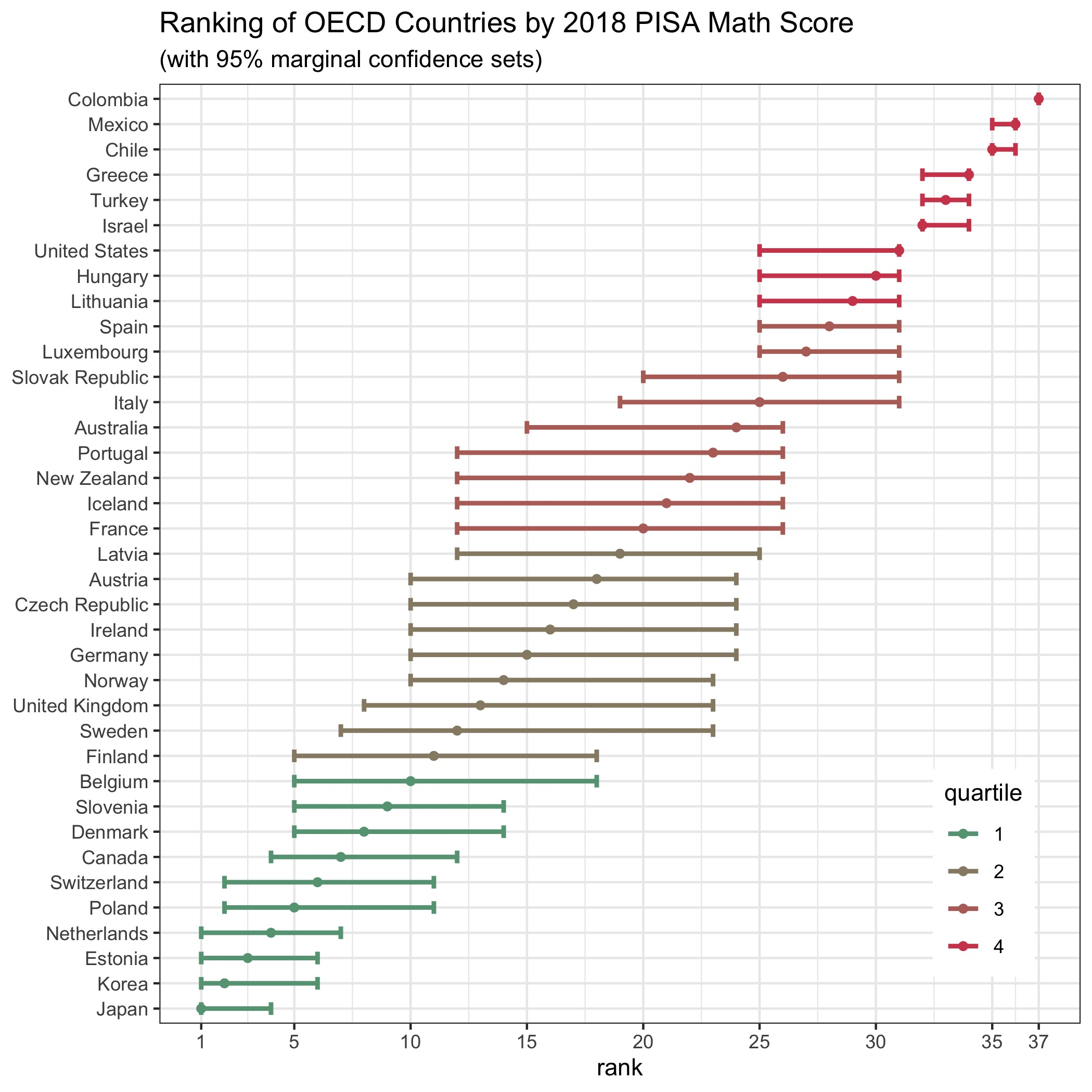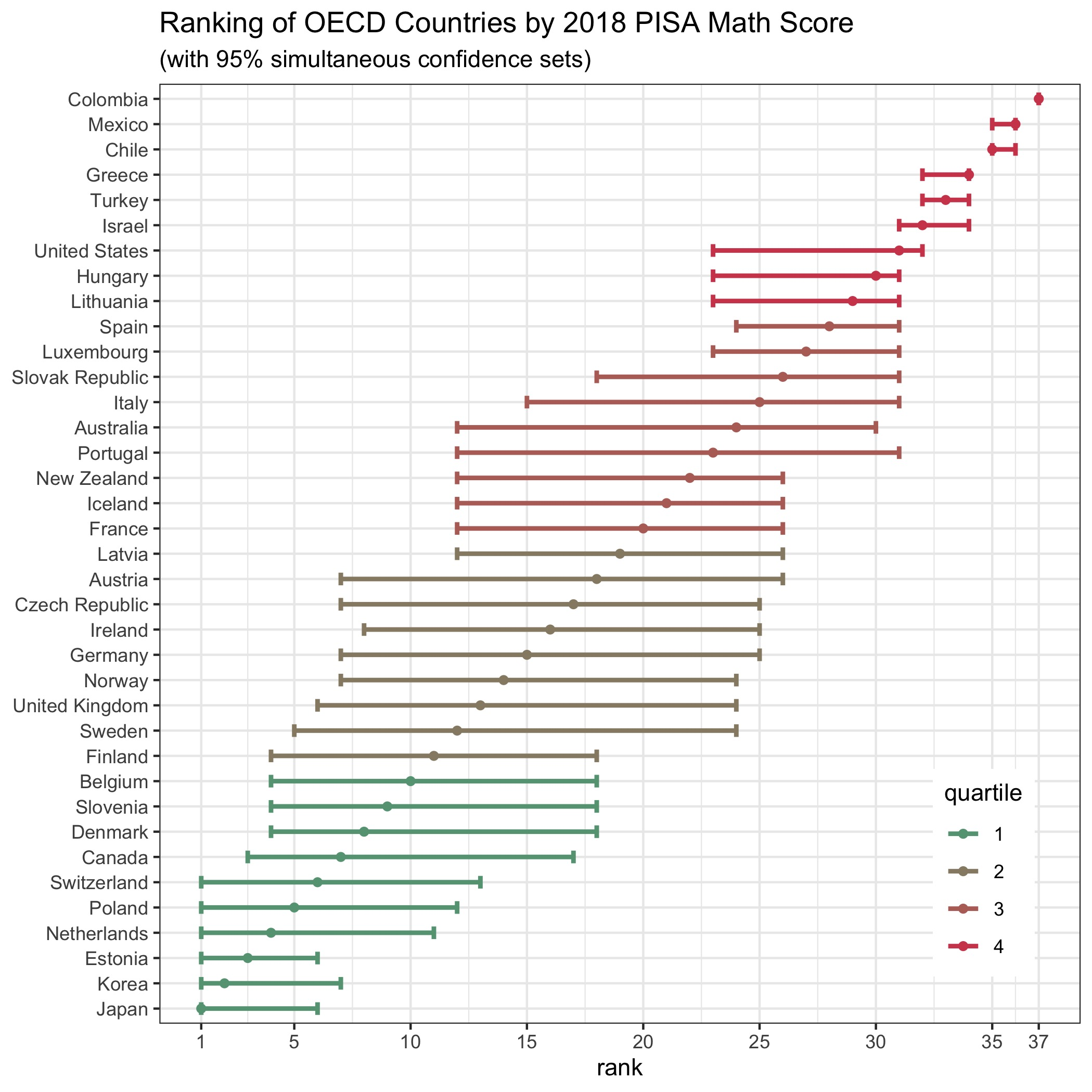The R package csranks implements confidence sets for ranks as in Mogstad, Romano, Shaikh, and Wilhelm (2020).

## Installation

Install the package devtools if it isn’t already:

install.packages("devtools")

Load the package devtools:

library("devtools")

Install the package csranks:

install_github("danielwilhelm/R-CS-ranks")

## Example: PISA Ranking

This example illustrates the computation of confidence sets for the ranking of countries according students’ achievements in mathematics. The following dataset contains data from the 2018 Program for International Student Assessment (PISA) study by the Organization for Economic Cooperation and Development (OECD), which can be downloaded at https://www.oecd.org/pisa/data/. Students’ achievement is measured by the average mathematics test scores for all 15-year-old students in the study.

First, load the package csranks and the data:

library(csranks)
attach(pisa)

The dataframe pisa looks like this:

print(pisa)

1        Australia      502.9646   1.795398      502.6317   1.634343   491.3600 1.939833
2          Austria      489.7804   2.777395      484.3926   2.697472   498.9423 2.970999
3          Belgium      498.7731   2.229240      492.8644   2.321973   508.0703 2.262662
4           Canada      517.9977   2.153651      520.0855   1.799716   512.0169 2.357476
5            Chile      443.5826   2.415280      452.2726   2.643766   417.4066 2.415888
6         Colombia      413.3230   3.052402      412.2951   3.251344   390.9323 2.989559
7   Czech Republic      496.7913   2.546069      490.2188   2.547851   499.4677 2.460662
8          Denmark      492.6370   1.937604      501.1299   1.795458   509.3984 1.735002
9          Estonia      530.1080   1.884569      523.0170   1.842242   523.4146 1.743602
10         Finland      521.8846   2.509515      520.0787   2.307102   507.3014 1.967920
11          France      492.9771   2.223662      492.6065   2.320979   495.4076 2.320214
12         Germany      502.9889   2.911501      498.2793   3.025408   500.0438 2.647083
13          Greece      451.6327   3.139630      457.4144   3.621000   451.3703 3.091228
14         Hungary      480.9117   2.329977      475.9867   2.250962   481.0826 2.319597
15         Iceland      475.0241   1.795502      473.9743   1.741511   495.1874 1.953083
16         Ireland      496.1136   2.214146      518.0785   2.244478   499.6329 2.198621
17          Israel      462.1966   3.618388      470.4152   3.670985   463.0345 3.498563
18           Italy      468.0117   2.429562      476.2847   2.438910   486.5899 2.780046
19           Japan      529.1354   2.593168      503.8560   2.670974   526.9733 2.471475
20           Korea      519.0073   2.802762      514.0523   2.940543   525.9330 3.121394
21          Latvia      487.2506   1.762796      478.6987   1.616856   496.1263 1.962767
22       Lithuania      482.0670   1.629237      475.8735   1.518276   481.1912 1.953282
23      Luxembourg      476.7694   1.220843      469.9854   1.125891   483.4215 1.097632
24          Mexico      419.2047   2.584009      420.4689   2.746279   408.8015 2.493226
25     Netherlands      503.3838   2.840622      484.7837   2.650864   519.2310 2.632278
26     New Zealand      508.4907   2.104634      505.7273   2.043143   494.4897 1.705943
27          Norway      490.4131   2.282508      499.4510   2.171849   500.9638 2.219045
28          Poland      511.0356   2.607236      511.8557   2.702458   515.6479 2.602085
29        Portugal      491.6773   2.773163      491.8008   2.428931   492.4874 2.684570
30 Slovak Republic      464.0476   2.278188      457.9840   2.234129   486.1649 2.555596
31        Slovenia      507.0065   1.250420      495.3456   1.232299   508.8975 1.363878
32           Spain      483.2520   1.552853            NA         NA   481.3926 1.462418
33          Sweden      499.4447   3.069711      505.7852   3.024772   502.3877 2.654251
34     Switzerland      495.2763   3.004997      483.9294   3.124322   515.3147 2.908004
35          Turkey      468.2996   2.013049      465.6317   2.171214   453.5078 2.260407
36  United Kingdom      504.6675   2.564415      503.9281   2.582591   501.7699 2.564428
37   United States      502.3800   3.317920      505.3528   3.568673   478.2447 3.235444

Consider ranking countries according to their students’ achievement in mathematics. The scores in math_score are estimates of these achievements. The countries’ ranks can be estimated using the function xrank():

math_rank <- xrank(math_score)
math_rank
 24 18 10  7 35 37 17  8  3 11 20 15 34 30 21 16 32 25  1  2 19 29 27 36  4 22 14  5 23 26  9 28 12  6 33 13 31

These are only estimated ranks as they are computed from estimates of achievements and therefore estimation uncertainty in the estimates translates to estimation uncertainty in the ranks. The following subsections discuss two different confidence sets for assessing such estimation uncertainty in ranks: marginal and simultaneous confidence sets. Explanations and details about the differences of these two confidence sets can be found in Mogstad, Romano, Shaikh, and Wilhelm (2020). The complete R script containing this example, as well as R scripts for PISA rankings by reading and science scores, can be found in the subdirectory examples/.

### Marginal Confidence Sets

The marginal confidence sets for the ranks are computed as follows:

CS_marg <- csranks(math_score, math_se, coverage=0.95, simul=FALSE, R=1000, seed=101)
math_rankL_marg <- CS_marg$L math_rankU_marg <- CS_marg$U

where math_rankL_marg and math_rankL_marg contain the lower and upper bounds of the confidence sets for the ranks. They can be plotted by

grid::current.viewport()

plotmarg <- plotranking(ranks=math_rank, L=math_rankL_marg, U=math_rankU_marg,
popnames=jurisdiction, title="Ranking of OECD Countries by 2018 PISA Math Score",
subtitle="(with 95% marginal confidence sets)", colorbins=4)

print(plotmarg)You can then save the graph by

ggplot2::ggsave("mathmarg.pdf", plot=plotmarg)

### Simultaneous Confidence Sets

The simultaneous confidence sets for the ranks are computed as follows:

CS_simul <- csranks(math_score, math_se, coverage=0.95, simul=TRUE, R=1000, seed=101)
math_rankL_simul <- CS_simul$L math_rankU_simul <- CS_simul$U

where math_rankL_simul and math_rankL_simul contain the lower and upper bounds of the confidence sets for the ranks. They can be plotted by

grid::current.viewport()

plotsimul <- plotranking(ranks=math_rank, L=math_rankL_simul, U=math_rankU_simul,
popnames=jurisdiction, title="Ranking of OECD Countries by 2018 PISA Math Score",
subtitle="(with 95% simultaneous confidence sets)", colorbins=4)

print(plotsimul)You can then save the graph by

ggplot2::ggsave("mathsimul.pdf", plot=plotsimul)